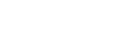Home » Jobs & Education » Education » X% of Y is the same as Y% of X, so for example 32% of 50 is the same as 50% of 32, which is 16.# X% of Y is the same as Y% of X, so for example 32% of 50 is the same as 50% of 32, which is 16.Nowdays, there is very high competition in all examinations, So merely knowing all the concepts is not going to take you through examination, There is a need to solve the Questions quickly, it comes through some tricks and lot of practice.This article will help you in solving some percentage questions quickly.

Statement: Is X% of Y same as Y% of X ?( pause for a moment and think, then proceed)

So, From equation 1 and 2 it is clear that X% of Y has exactly same value as Y% of X.

The above statement reduces our calculation in many percentage problems.

Solution: Since we know, X% of Y and Y% of X are same.

If you are good in converting percentage into fraction, and you know that X% of Y is same as Y% of X.You can easily reduce calculation in many percentage problems.

Summary Always try to use the relation X% of Y= Y% of X.

Solution: In this question it does not look directly that here we can apply X% of Y = Y% of X.

So, 42% of 250 + 25% of 420= 25% of 420 + 25% of 420 = 50% of 420 = 1/2* 420 = 210 Ans. Now its your turn, Solve few mock Questions..

Try to do all the questions mentally without using pen, if it does not get solved mentally then only use pen & paper.

## [Aptitude] Percentage: X% of Y is same as Y% of X.

Nowdays, there is very high competition in all examinations, So merely knowing all the concepts is not going to take you through examination, There is a need to solve the Questions quickly, it comes through some tricks and lot of practice.This article will help you in solving some percentage questions quickly.

Percentage to Fraction Conversion

Let us Begin:

Statement: Is X% of Y same as Y% of X ?( pause for a moment and think, then proceed)

Let us verify above statement.

X% of Y = X/100 * Y= X*Y/100 — (1)

&

Y% of X = Y/100 * X= Y*X/100 — (2)

So, From equation 1 and 2 it is clear that X% of Y has exactly same value as Y% of X.

The above statement reduces our calculation in many percentage problems. Let us take an example.

Ques: Calculate 32 % of 50.

Solution: Since we know, X% of Y and Y% of X are same.

So, 32% of 50 = 50% of 32.

Now there is nothing left to calculate as you all know,

50% = 1/2

So, 50% of 32= 1/2*32= 16.

If you are good in converting percentage into fraction, and you know that X% of Y is same as Y% of X.You can easily reduce calculation in many percentage problems.

Summary Always try to use the relation X% of Y= Y% of X. Through practice you can master your brain, which will automatically convert it, and calculation will become easier for you.

Let us try another problem.

Ques: what is the value of

45% of 280 + 28% of 450.

Solution: Any one can solve above expressioni, But our purpose is to solve it quickly.

Look at the second term,

28% of 450 can be written as 45% of 280. ( those who doubt everything can solve and varify)

Now, our expression becomes,

45% of 280 + 45% of 280= 90% of 280= (100% of 280)-( 10% of 280)= 280-28= 252 Ans.

( fraction equivalent of 100% =1 & fraction equivalent of 10% =1/10)

Question: 42% of 250 + 12.5% of 840.

Solution: In this question it does not look directly that here we can apply X% of Y = Y% of X.

Look at the second expression.

12.5% of 840 = 25% of 420 ( as 25% of 100 is same as 50% of 50)

Now our expression becomes.

42% of 250 + 25% of 420

Since 42% of 250=25% of 420.

So, 42% of 250 + 25% of 420= 25% of 420 + 25% of 420 = 50% of 420 = 1/2* 420 = 210 Ans.

Now its your turn, Solve few mock Questions.. Try to do all the questions mentally without using pen, if it does not get solved mentally then only use pen & paper.

Mock Questions

Q1) 68% of 25 = ?

A] 15

B] 16

C] 17

D] 18

Q2) 38% of 50 = ?

A] 16

B] 17

C] 18

D] 19

Q3] 55% of 460 + 46% of 550=?

A] 414

B] 424

C] 496

D] 506

Q4) 48% of 400 + 20% of 960= ?

A] 364

B] 384

C] 416

D] 436

Enroll for Online Courses By RaMo (99.99%iler CAT) and give A boost to your preparation.